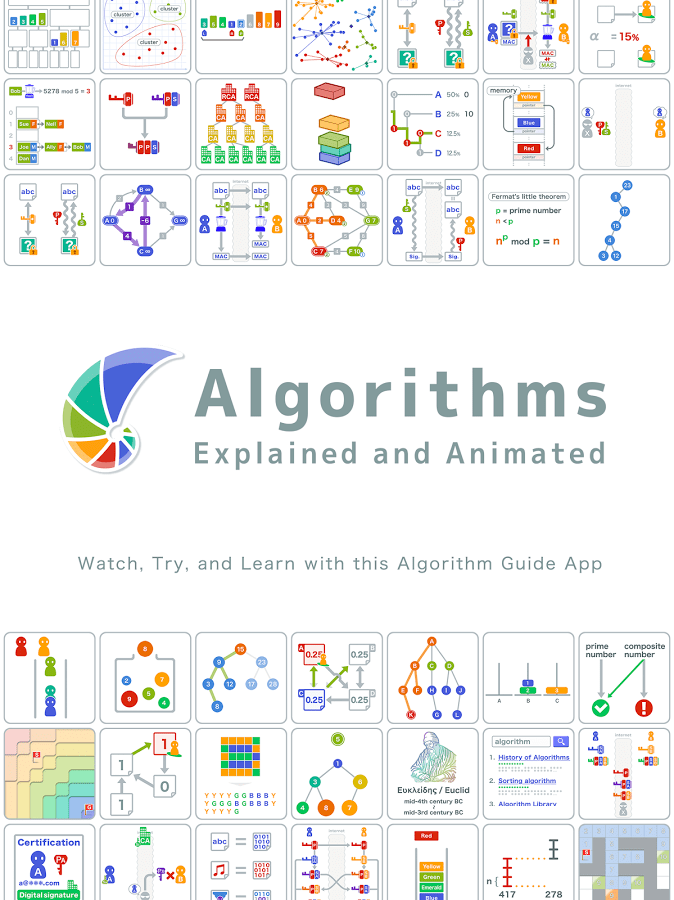# Algorithms

Etymology: late 17th century (denoting the Arabic or decimal notation of numbers): variant (influenced by Greek arithmos ‘number’) of Middle English algorism, via Old French from medieval Latin algorismus . The Arabic source, al-Ḵwārizmī ‘the man of Ḵwārizm’ (now Khiva), was a name given to the 9th-century mathematician Abū Ja‘far Muhammad ibn Mūsa, author of widely translated works on algebra and arithmetic.

## In Mathematics

In mathematics and computer science, an algorithm is a self-contained sequence of actions to be performed. Algorithms perform calculation, data processing, and/or automated reasoning tasks.

An algorithm is an effective method that can be expressed within a finite amount of space and time and in a well-defined formal language for calculating a function. Starting from an initial state and initial input (perhaps empty), the instructions describe a computation that, when executed, proceeds through a finite number of well-defined successive states, eventually producing “output” and terminating at a final ending state. The transition from one state to the next is not necessarily deterministic; some algorithms, known as randomized algorithms, incorporate random input.

### The concept of algorithm

The concept of algorithm has existed for centuries; however, a partial formalization of what would become the modern algorithm began with attempts to solve the Entscheidungsproblem (the “decision problem”) posed by David Hilbert in 1928.

Subsequent formalizations were framed as attempts to define “effective calculability” or “effective method”; those formalizations included the Gödel–Herbrand–Kleene recursive functions of 1930, 1934 and 1935, Alonzo Church’s lambda calculus of 1936, Emil Post’s “Formulation 1” of 1936, and Alan Turing’s Turing machines of 1936–7 and 1939. Giving a formal definition of algorithms, corresponding to the intuitive notion, remains a challenging problem. Read more at wikipedia

### Example by Standford Education#### Interesting Articles

Algorithms Explained & Animated by Google Play